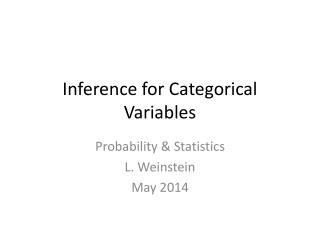DownloadDownload PresentationInference for Categorical Variables

# Inference for Categorical Variables

Télécharger la présentation## Inference for Categorical Variables

- - - - - - - - - - - - - - - - - - - - - - - - - - - E N D - - - - - - - - - - - - - - - - - - - - - - - - - - -
##### Presentation Transcript

1. Inference for Categorical Variables Probability & Statistics L. Weinstein May 2014

2. Testing a Claim with Categorical Data • Three tests: • Goodness of Fit TestDoes the distribution of the categorical variable fit an expected model? • Test for Homogeneity of PopulationsDoes each population have the same distribution for this variable? • Test for Association / IndependenceAre two categorical variables associated?

3. Goodness of Fit Test State: Is the distribution of <your variable here> different from the expected distribution of <be specific here>? The distribution is the same as expected for all categories The distribution is the different than expected for at least one category Test at significance level <choose a level>

4. Goodness of Fit Test Plan: Use a Goodness of Fit test Conditions: • Sample is randomly selected from population • All expected counts are at least 5 • Sample observations are independent; that is, if sampling without replacement, sample size is not more then 10% of the population size.

5. Goodness of Fit Test To conduct the test in Minitab, summarize the data by category and put this in one column. If equal counts are expected, this is enough. If something other than equal counts are expected, make a column of expected counts. Then run Stat>Tables>Chi-Square Goodness of Fit Test in Minitab.

6. Goodness of Fit Test Enter the column names for Observed Counts, Category names, and Proportions specified by historical counts (this is your expected counts list):

7. Goodness of Fit Test Do: <Include Minitab results of chi-square test here> <Indicate the value of the test statistics, , and the P-value of the test.>

8. Goodness of Fit Test Conclude: <Compare your P-Value to your significance level. Based on this comparison, either reject or fail to reject the null hypothesis. Conclude, or do NOT conclude, the alternative hypothesis in words.>

9. Test for Homogeneity State: Is the distribution of <your variable here> different for the populations <be specific here>? The distribution is the same for all populations The distribution is the different for at least one category Test at significance level <choose a level>

10. Test for Homogeneity Plan: Use a Test for Homogeneity Conditions: • Samples are randomly selected from each population • All expected counts are at least 5 • Sample observations are independent; that is, if sampling without replacement, each sample size is not more then 10% of that population size.

11. Test for Homogeneity To conduct the test in Minitab, make a column of the summarized distribution of the variable for each population. Then run Stat>Tables>Chi-Square Test (2-way table) in Minitab.

12. Test for Homogeneity Enter the column names for each population:

13. Test for Homogeneity Do: <Include Minitab results of chi-square test here> <Indicate the value of the test statistics, , and the P-value of the test.>

14. Test for Homogeneity Conclude: <Compare your P-Value to your significance level. Based on this comparison, either reject or fail to reject the null hypothesis. Conclude, or do NOT conclude, the alternative hypothesis in words.>

15. Test for Independence State: Is there an association between <categorical variable one> and <categorical variable two>? There is no association between the variables (they are independent). There is an association between the variables (they are NOT independent. Test at significance level <choose a level>

16. Test for Independence Plan: Use a Test for Independence / Association Conditions: • Sample is randomly selected from population • All expected counts are at least 5 • Sample observations are independent; that is, if sampling without replacement, sample size is not more then 10% of the population size.

17. Test for Independence To conduct the test in Minitab, make a two-way table summarizing the observed counts for each category of the two variables. Then run Stat>Tables>Chi-Square Test (2-way table) in Minitab.

18. Test for Independence Enter the column names that contain the summarized data:

19. Test for Independence Do: <Include Minitab results of chi-square test here> <Indicate the value of the test statistics, , and the P-value of the test.>

20. Test for Independence Conclude: <Compare your P-Value to your significance level. Based on this comparison, either reject or fail to reject the null hypothesis. Conclude, or do NOT conclude, the alternative hypothesis in words.>